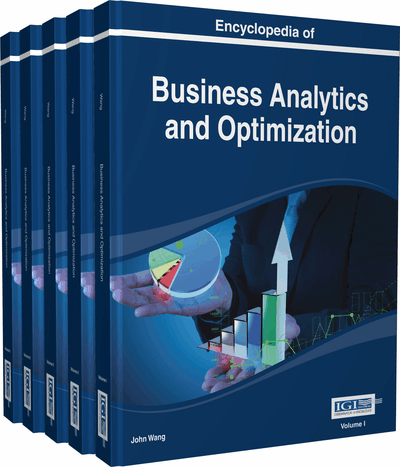# FGP Model for Emission-Economic Power Dispatch

Mousumi Kumar (Alipurduar College, India) and Bijay Baran Pal (University of Kalyani, India)
DOI: 10.4018/978-1-4666-5202-6.ch087

Top

## Introduction

Electricity generation is the process of generating electric power from natural energy sources. In early 1830s, the famous British scientist Michael Faraday discovered the fundamental principles of electricity generation.

In most of the cases, electricity is generated from power plants by burning fossil-fuels: coal, oil and natural gas, which produce more pollutions than any other single industry and vastly contribute various impacts to the living environment. However, generation of electric power is increasing in an alarming rate to meet demand in the recent years by ignoring ill effects of pollution to the earth’s environment. Therefore, proper planning for economic power generation as well as control of environmental pollution are inevitable ones in the context of generation of electricity from power plants.

The general mathematical programming (MP) model for optimal power generation was introduced by Dommel and Tinney (1968). A Comprehensive Survey on environmental power dispatch models developed from 1960s to 1970s was first surveyed by Happ (1977). Since an emission-economic power dispatch (EEPD) problem is multiobjective in nature, the goal programming (GP) approach (Ignizio, 1976), based on the satisficing philosophy (coined by Noble Laureate H. A. Simon) (Simon, 1945), as a robust tool for multiobjective decision analysis, has been successfully implemented to power generation problems (Nanda, Kothari, & Lingamurthy,1988) in the past. The field of chance constrained programming (CCP) (Charnes & Cooper, 1959) has been studied extensively and applied to various real-life problems (Keown & Taylor, 1980) including EEPD problem (Dhillon, Parti, & Kothari, 1993).

However, in most of the practical decision situations, it has been observed that model parameters associated with such problems are often imprecise in nature. The most prominent approach for decision analysis in an uncertain (not precise) environment is fuzzy programming (FP) (Tanaka, Okuda, & Asai, 1974), which is based on the theory of fuzzy sets (Zadeh, 1965).

Again, fuzzy goal programming (FGP) (Pal, Moitra, & Maulik, 2003) as an extension of conventional GP has also appeared as a robust tool to make flexible decision (Pal, Kumar, & Sen, 2009) in fuzzy environment. The FGP approach to EEPD problems has been studied (Pal, Chakraborti, & Biswas, 2011) in the recent past. But, the deep study on the potential use of such an approach is thin and yet to be widely circulated in the literature. Further, in most of the previous studies in this area, only two objectives, minimization of production cost and environmental-emission have been taken into account. But, consideration of other objectives inherent to an EEPD problem is rare in the literature.

In this chapter, minimization of transmission-loss as a prominent one along with the other two objectives stated previously is considered for modeling and solving EEPD problems. In the model formulation, membership goals of the membership functions associated with fuzzily described objectives of the problem are defined by introducing highest membership value (unity) as aspiration level and introducing under- and over-deviational variables to each of the membership functions. Again, the inherent nonlinear objective functions and constraints are transformed into their linear forms to solve the problem by employing linear FGP methodology.

In the solution process, sensitivity analysis with variations of priority structure of model goals is performed and then the Euclidean distance function is used to identify the appropriate priority structure to achieving the most satisfactory decision for power generation in the decision situation.

The effectiveness of the proposed is illustrated by standard IEEE 30-bus 6-generator test system. To expound the potential use of the approach, the model solution is compared with the solutions obtained by using other approaches studied previously.

## Key Terms in this Chapter

Emission-Economic Power Dispatch: Emission-economic power dispatch is a general thermal power plant optimization problem with the objectives of minimizing both the power generation cost and environmental emission.

Goal Programming: Goal programming is an optimization technique to solve problems with multiplicity of objective in a decision making horizon.

Non-Linear Programming: Nonlinear programming is concerned with solving a class of mathematical programming problems in which the objective and / or system constraints are nonlinear in nature.

Fuzzy Goal Programming: Fuzzy goal programming is an extension of conventional goal programming to solve multiobjective problems with imprecisely defined model parameters in a decision making environment.

Taylor Series Approximation: Taylor series approximation is a mathematical approach for linearization of nonlinear functions, mainly the problems with quadratic functions.

Stochastic Programming: Stochastic programming is an optimization technique for solving problems with uncertain data, where probability distributions of model parameters are involved in a decision environment.

Fuzzy Programming: Fuzzy programming is an optimization method for solving problems with imprecisely defined model parameter sets rather than crisp/ probabilistic in the traditional sense.

## Complete Chapter List

Search this Book:
Reset